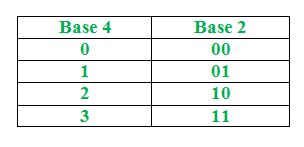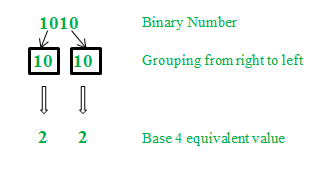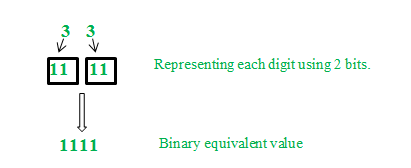# Conversion of Binary number to Base 4 system

We all know that,=.
In other words, single digit in base 4 can be represented using 2 digits in base 2. Base 2 has digits 0 and 1. Similarly base 4 has digits 0, 1, 2 and 3.Algorithm for Binary to Base 4 Conversion

1. Beginning from right, group the digits of binary (base 2) number into group of two.
2. Write the base 4 equivalent value corresponding to the each group.

Example: Convert the binary number 1010 to base 4.Therefore the base 4 equivalent of binary number 1010 is 22.

Now, Algorithm for Base 4 to Binary Conversion: Represent each digit in the base 4 number using 2 bits.

Example: Convert the base 4 number 22 to binary.Therefore the binary equivalent of base 4 number 22 is 1010.

Practice Questions:
(1) Convert the binary number 111000 to base 4.
(2) Convert the binary number 100100 to base 4.
(3) Convert the binary number 1001001111 to base 4.
(4) What is the binary equivalent of base 4 number 3333.
(5) What is the binary equivalent of base 4 number 111303.

```(1) 320
(2) 210
(3) 21033
(4) 11111111
(5) 10101110011```

Attention reader! Don’t stop learning now. Get hold of all the important CS Theory concepts for SDE interviews with the CS Theory Course at a student-friendly price and become industry ready.

My Personal Notes arrow_drop_upCheck out this Author's contributed articles.

If you like GeeksforGeeks and would like to contribute, you can also write an article using contribute.geeksforgeeks.org or mail your article to contribute@geeksforgeeks.org. See your article appearing on the GeeksforGeeks main page and help other Geeks.

Please Improve this article if you find anything incorrect by clicking on the "Improve Article" button below.